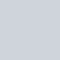# sorting 簡單說明 (記憶用)

Selection sort (選擇排序)先完成的排序是「當前index」，從當前往後搜尋全部，找最小
Insertion sort (插入排序)每一層 loop 會完成的是「當前 index」之前的所有排序，也就是說，for-loop 的外層代表處理完成的 index，而內層處理插入當前的哪個位置
Bubble sort (氣泡排序)不斷交換的排序，已交換len=4為例，比較 「(1,2),(2,3,(3,4)) / (1,2),(2,3),(3,4) / (1,2),(2,3),(3,4)」 (共 3組 x 3輪)
Quick sort (快速排序)1.先挑選一個基準 (pivot)，經常選最後一個值，後續的數值與基準比大小，建立two pointer，比小往左、比大往右，「停止條件：two pointer 交錯」 / 2.每一輪完成一次「對基準排序」後，將基準擺在中間 / 3. 依據 「divide and conquer (O(logn))」 的概念，完成剩餘的左右兩側(partition)的基準排序 (partition = [0, pivot-1], [pivot], [pivot+1, n-1]) 「停止條件：代表 front,end 的 two pointer 交錯
Merge sort (合併排序)一樣也是「divide and conquer (O(logn))」 的概念，1. 將 array 拆成 n/2，n/2再拆兩個n/4… 2. 拆到剩餘 2 or 1(種子)，開始合併，合併時只需要看如何已排序的兩數列如何交錯即可(O(m+n))，使用 two pointer
Heap sort (堆積排序)Complete Binary Tree /Max Heap (root max) / MaxHeapify() = 自上而下調整矩陣 BuildMaxHeap() 1. 最後一個位置換到第一個 / 2.最後一個消失(取得 Max) 3. 自上而下的 MaxHeapify()

# sorting 整理

BestWorstAverageSpace Complexity
Selection sortO(n^2)O(n^2)O(n^2)O(1)，無新增矩陣等級的空間使用
Insertion sortO(n) 當數列已經是從小到大的O(n^2)O(n^2)|
Bubble sort|
|

# 特殊情況

• bubble sort early break: 如果某一回合沒有 swap 發生，直接結束

• insertion sort : 恰好小至大，免做「插入動作 O(n)

• quick sort can not divide well: 如果一個矩陣從小到大或從大到小，因為沒辦法好好發揮 divide and conquer 的效果，O(m+n)*O(n) 的 m=0, n=n (類似概念) = O(n^2)

# 說明

(資料太整齊, pivot 產生不了 Divide and conquer的效果)# Reference